# Calculating the Number of Time Periods (n)

If we know the present value (PV), the future value (FV), and the interest rate per period of compounding (i), the future value factors allow us to calculate the unknown number of time periods of compound interest (n). Calculations #5 through #8 illustrate how to determine the number of time periods (n).

### Calculation #5

An airplane ticket costs \$500 today and it is expected to increase at a rate of 5% per year compounded annually. Determine the number of years it will take for the \$500 airplane ticket to have a future cost of \$700.

The following timeline plots the variables that are known and unknown: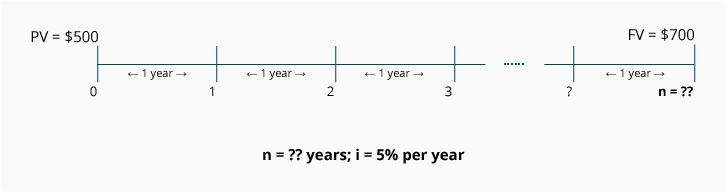Because the rate of increase is compounded annually, we use the given annual rate of 5%. The answer (n) will be stated in annual time periods (years).

Calculation using the FV of 1 Table: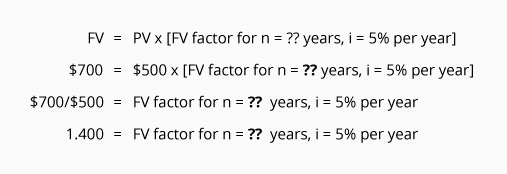To finish solving the equation, we search only the i = 5% column of the FV of 1 Table for the future value factor that is closest to 1.400. In this case, the factor we find is 1.407, and we see it is located in the row where n = 7. This tells us that it will take approximately 7 annual time periods (7 years) for an airplane ticket to go from its present cost of \$500 to the future cost of \$700.

### Calculation #6

Lorenzo put \$600 today in an account that earns an annual rate of 8% compounded semiannually. How many years will it take for Lorenzo's single investment of \$600 to have a future value of \$900?

The following timeline plots the variables that are known and unknown: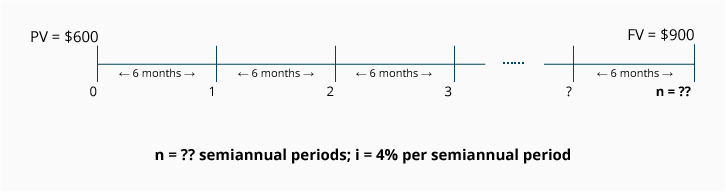Because the interest is compounded semiannually, we converted the annual interest rate of 8% to the semiannual rate of 4%.

Calculation using the FV of 1 Table: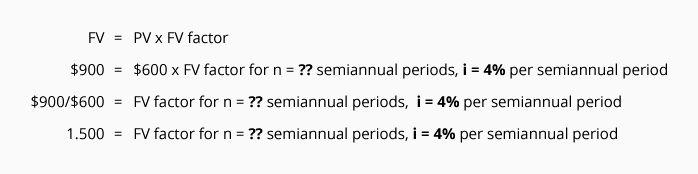To finish solving the equation, we search only the 4% column of the FV of 1 Table for the future value factor that is closest to 1.500. In this case, the factor we find is 1.480, and we see it is located in the row where n = 10. This means it will require 5 years (10 semiannual time periods divided by 2 semiannual periods in each year) for Lorenzo's \$600 to reach a future value of \$900.

### Calculation #7

Nancy invests a sum of \$700 at a fixed rate of 8% per year with quarterly compounding. How many years will it take her \$700 investment to reach a future value of \$1,000?

The following timeline plots the variables that are known and unknown: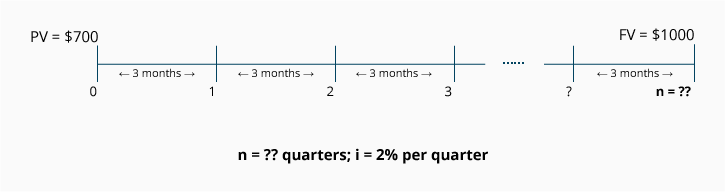Because the interest is compounded quarterly (every 3 months), the annual interest rate is converted to 2% per quarter.

Calculation using the FV of 1 Table: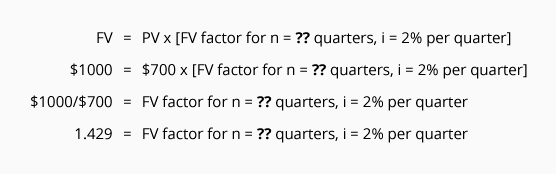To finish solving the equation, we search only the 2% column of the FV of 1 Table for the future value factor that is closest to 1.429. In this case, the factor is 1.428, and we see it is located in the row where n = 18.

To convert n = 18 quarters to years, we simply divide the 18 quarters by 4, the number of quarterly periods in a year. The answer is that it will take approximately 4.5 years for Nancy's \$700 investment to reach a future value of \$1,000.

### Calculation #8

You invest \$787 today in an account that will return an annual interest rate of 12% with interest compounded monthly. How many years will it take for the \$787 investment to have a future value of \$1,000?

The following timeline plots the variables that are known and unknown: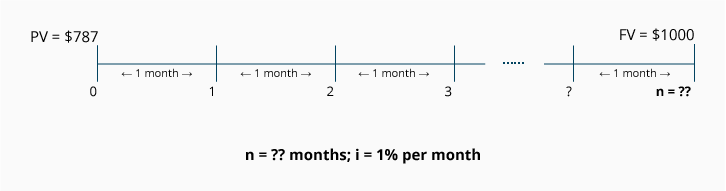Because the interest is compounded monthly, we convert the annual rate of 12% to i = 1% per month.

Calculation using the FV of 1 Table: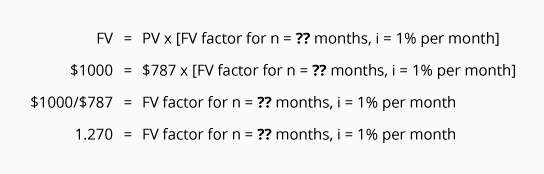To finish solving the equation, we search only the i = 1% column in the FV of 1 Table for the FV factor that is closest to 1.270. In this case, there is a factor of exactly 1.270, and it is located in the row where n = 24.

Since n = 24 monthly time periods, we need to divide the 24 months by 12 months in a year in order to get the answer in years. It will take approximately 2 years for your \$787 investment to reach a future value of \$1,000.